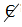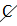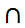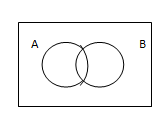# Set Language And Notation

### Set:

A set is a collection of objects, things or symbols which are clearly identified.

#### 🚚 Delivering all over PAKISTAN 🇵🇰 & ✈️Internationally 🌐

The individual objects in the set are called the elements or members of the set.

Elements may be specified in two ways:

• By listing the elements
• By description

E.g.

### 😃 Relax your Mind From Studying and WATCH this Beautiful Painting! 👇

Listing                                                             Description

{2, 4, 6, 8, 10}                                                  The set of even numbers between 1 and 11

{a, e, i, o,u}                                                      The set of vowels in the alphabet

{} These braces stand for the word “the set of”

e.g.  {Even numbers between 1 and 11}

### Naming sets and number of members in a set:

Usually, we use Capital letters to denote a set and small letters to denote members of the set.

n ( ) indicates the total number of members in a set.

E.g.

A= {1, 3, 5, 7, 9, 11}      n (A) = 6

B= {2, 4, 6, 8}         n (B) = 4

### Membership of the set:

Є is an element of  (is a member of ) (belongs to)is not an element of  (is not a member of) ( does not belongs to)

E.g.

A = {1, 3, 5, 7, 9, 11}

### Finite Sets:

Set in which all the elements can be listed.

A= {1,3,5,7,9}  n(A) =5

B= {days of the week beginning with T}  n(B) = 2

### Infinite Sets:

Sets in which it is possible to list all the members of a set.

C= {2, 4, 6, 8,10….}

E={x:x is a natural number}

### Universal Sets: (Ƹ)

The set which contains all the elements.

All proper subsets formed within the universal set draw their elements from the available elements of the universal sets.

### Complement Of a Set: (A`)

(Ƹ element – A element} = A`

If Ƹ = {2, 3, 5, 7, 11, 13}  and A = {2, 3, 7, 13}

A= {5, 11}

### Equal Sets:(C)

Two sets A and B are said to be equal if and only they have exactly the same elements.

Two equal sets are also subsets (denoted by C) of each other.

A={2,4,6,8}           B={8,6,2,4}

Then

A=B      B=A      A C B  B C A

### Subsets:

When each member of a set A is also a member of a set B, then A is a subset of B.

C is a subset of :When two sets have  exactly same elements or elements in the first set are also elements in the   second set.is not a subset of: There is at least one element in the first set that does not belong to the second set.

### Proper Subset:

When each member of a set A is also a member of a set B, but set B has MORE elements than set A, then set A is a proper subset of B, denoted by “A C B”. Therefore, in this case set B is not a proper subset of A (BA)

C is a proper subset of: When each element in the first set also belongs to the second set, but the second set has more elements than the first set.

C is not a proper subset: When there is at least, one element in the first set that does not belong to the second set.

E.g. A = {1, 5, 9}     B = {1, 3, 5, 9}

### Empty Set: ({} or)

A set which contains NO elements

An empty set in a subset of any set.

### Intersection Of Sets: ()

Common elements in different sets.

A= {1,2,3,4,5,6}

B= {2,4,8,10}

Union Of Sets: U

The Union of set A and set B is the set of all elements which are in A, or in B, or in both A and B. It is denoted by ‘A U B’ and is read as “the union of A and B”.

A= {1,3,4}    B={6,7,8}

A U B = {1,3,4,6,7,8}

### Disjoint Sets:

If the two sets have No element in common then the two sets are called disjoint.

The intersection of two disjoint sets is null or empty.

e.g. A = {1,3,5,7}    and     B = {2,4,6,8,9}

De Morgans Law:### Venn Diagram:

In a venn diagram, we use a rectangle to denote a universal set Ƹ and a loop such as circle or an oval to represent any set in Ƹ .Examples of Venn Diagrams:### 😃 Relax your Mind From Studying and WATCH this Beautiful Painting! 👇1.2.